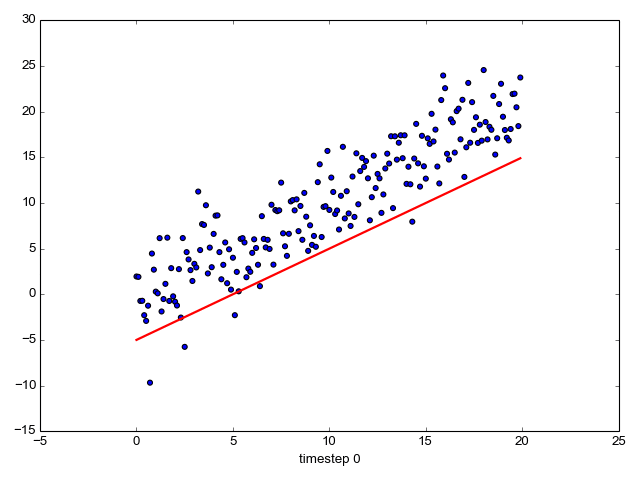• 源码在此：我将一张caixukun打篮球的动图处理成字符动图 # 将gif动图转gif字符动图 from PIL import Image,ImageSequence,ImageDraw import numpy as np import os import imageio #读取动图 img=Image.open('d:/....
效果图放不了了，没想到gif图内存很大，可惜了，不能一睹坤坤风采。

源码在此：我将一张caixukun打篮球的动图处理成字符动图

# 将gif动图转gif字符动图
from PIL import Image,ImageSequence,ImageDraw
import numpy as np
import os
import imageio
#读取动图
img=Image.open('d:/111.jpg')
#调整大小
img.resize((150,150))
#宽高
w,h=img.size
#读取动图各个帧
iter=ImageSequence.Iterator(img)
#拷贝，并转成RGB图
imgs=[frame.copy().convert('RGB') for frame in iter]
# imgs=[frame.copy().convert('L') for frame in iter]
#设定字符
s="\$@B%8&WM#*oahkbdpqwmZO0QLCJUYXzcvunxrjft/\|()1{}[]?-_+~<>i!lI;:,\"^'. "
#将像素映射为字符
def pixel_to_char(p,alpha=256):
r=p
g=p
b=p
if alpha==0:
return ""
length=len(s)
gray=int(0.2126*r+0.7152*g+0.0722*b)
unit=(256.+1)/length
return s[int(gray/unit)]
#将图片转为字符图
def changeImg(img,index):
#图像像素数组
# data=np.array(img)
#开始创建字符图了
new_img=Image.new('1',(2*w,2*h),color=255)#白色背景
draw=ImageDraw.Draw(new_img)
#高为行，宽为列，每隔5个取一次像素
for i in range(0,h,5):
for j in range(0,w,5):
ch=pixel_to_char(img.getpixel((j,i)))
#松散一些较好看
draw.text((2*j,2*i),ch)
#保存字符图
new_img.save('d:/caixukun/%d.png'%index)

#字符图存储
idx=0
for frame in imgs:
changeImg(frame,idx)
idx+=1

# 创建gif
path='d:/caixukun/'
imgs=[]
for file in os.listdir(path):
imgs.append(imageio.imread(os.path.join(path,file)))
# 图片保存为gif
imageio.mimsave('d:/caixukun.gif',imgs,'GIF',duration=0.04)


展开全文• Python 动图 动画制作 —— moviepy matplotlib animation
分享一下我老师大神的人工智能教程！零基础，通俗易懂！http://blog.csdn.net/jiangjunshow也欢迎大家转载本篇文章。分享知识，造福人民，实现我们中华民族伟大复兴！                       进入命令行界面（windows ⇒ cmd），下载安装，pip install moviepy0. figure 的成员函数# 创建 figurefig, ax = plt.subplots()fig = plt.figure(figsize(6, 8))# 成员函数fig.set_tight_layout(True)fig.get_dpi()fig.get_size_inches() 123456781. 使用 moviepyfrom moviepy.video.io.bindings import mplfig_to_npimageimport moviepy.editor as mpyf = plt.figure(figsize=(8, 8))# 接受一个时间参数，def make_frame_mpl(t):    ...    return mplfig_to_npimage(f)                # 所有的图像都在同一个figure上animation = mpy.VideoClip(make_frame_mpl, duration=5)animation.write_gif("animation-94a2c1ff.gif", fps=20)        # 这样其实就可以做一个动态图出来了；12345678910111213142. 使用 matplotlib.animationimport matplotlib.pyplot as pltfrom matplotlib.animation import FuncAnimationimport numpy as npimport seabornfig, ax = plt.subplots()x = np.arange(0, 20, .01)ax.scatter(x, x+np.random.normal(0, 3, len(x)))line, = ax.plot(x, x-5, 'r-', lw=2)# i => ith framedef update(i):    line.set_ydata(x-5+i)    ax.set_xlabel('frame {0}'.format(i))    return line, axif __name__ == '__main__':    animation = FuncAnimation(fig, update, frames=np.arange(0, 20), interval=200)        # 200ms 的间隔，相当于 5fps，一秒 5 帧    plt.show()1234567891011121314151617181920212223如果想要保存 animation，使得 gif 足够小，可在保存时，设置足够小的 dpi，以及在创建 fig 时，figsize 也设置为尽可能得小。animation.save('line.gif', dpi=80, writer='imagemagick')1            给我老师的人工智能教程打call！http://blog.csdn.net/jiangjunshow展开全文• 进入命令行界面（windows ⇒ cmd），下载安装， pip install moviepy from moviepy.video.io.bindings import mplfig_to_npimage import moviepy.editor as mpy

进入命令行界面（windows ⇒ cmd），下载安装，pip install moviepy

0. figure 的成员函数

# 创建 figure
fig, ax = plt.subplots()
fig = plt.figure(figsize(6, 8))

# 成员函数
fig.set_tight_layout(True)
fig.get_dpi()
fig.get_size_inches() 

1. 使用 moviepy

from moviepy.video.io.bindings import mplfig_to_npimage
import moviepy.editor as mpy

f = plt.figure(figsize=(8, 8))

# 接受一个时间参数，
def make_frame_mpl(t):
...
return mplfig_to_npimage(f)
# 所有的图像都在同一个figure上

animation = mpy.VideoClip(make_frame_mpl, duration=5)
animation.write_gif("animation-94a2c1ff.gif", fps=20)
# 这样其实就可以做一个动态图出来了；

2. 使用 matplotlib.animation

import matplotlib.pyplot as plt
from matplotlib.animation import FuncAnimation
import numpy as np
import seaborn

fig, ax = plt.subplots()

x = np.arange(0, 20, .01)
ax.scatter(x, x+np.random.normal(0, 3, len(x)))
line, = ax.plot(x, x-5, 'r-', lw=2)

# i => ith frame
def update(i):
line.set_ydata(x-5+i)
ax.set_xlabel('frame {0}'.format(i))
return line, ax

if __name__ == '__main__':

animation = FuncAnimation(fig, update, frames=np.arange(0, 20), interval=200)
# 200ms 的间隔，相当于 5fps，一秒 5 帧
plt.show()

如果想要保存 animation，使得 gif 足够小，可在保存时，设置足够小的 dpi，以及在创建 fig 时，figsize 也设置为尽可能得小。

animation.save('line.gif', dpi=80, writer='imagemagick')
展开全文• 其实我们还能让可视化图形逼格更高一些，今天就分享一下如何让可视化秀起来：用Python和matplotlib制作GIF图表。假如电脑上没有安装ImageMagick，先去这里按照自己的电脑系统下载对应版本，大家也可以通过脚本之家...
前言之前我们分享过用Python进行可视化的9种常见方式。其实我们还能让可视化图形逼格更高一些，今天就分享一下如何让可视化秀起来：用Python和matplotlib制作GIF图表。假如电脑上没有安装ImageMagick，先去这里按照自己的电脑系统下载对应版本，大家也可以通过脚本之家下载：https://www.jb51.net/softs/140766.html，如果我们想用matplotlib的save方法渲染GIF动图，就需要安装ImageMagick。下图是我们制作的一个动图示例：有两点需要注意： 图表中的散点不会动，会动的是直线。 X轴标题每一帧都在变化。下面是我们制作上面GIF图的代码：import sysimport numpy as npimport matplotlib.pyplot as pltfrom matplotlib.animation import FuncAnimationfig, ax = plt.subplots()fig.set_tight_layout(True)# 询问图形在屏幕上的大小和DPI（每英寸点数）# 注意当把图形保存为文件时，需要为此单独再提供一个DPIprint('fig size: {0} DPI, size in inches {1}'.format(fig.get_dpi(), fig.get_size_inches()))# 绘制一个保持不变（不会被重新绘制）的散点图以及初始直线x = np.arange(0, 20, 0.1)ax.scatter(x, x + np.random.normal(0, 3.0, len(x)))line, = ax.plot(x, x - 5, 'r-', linewidth=2)def update(i):label = 'timestep {0}'.format(i)print(label)# 更新直线和轴（用一个新X轴标签）# 以元组形式返回这一帧需要重新绘制的物体line.set_ydata(x - 5 + i)ax.set_xlabel(label)return line, axif __name__ == '__main__':# 会为每一帧调用Update函数# 这里FunAnimation设置一个10帧动画，每帧间隔200msanim = FuncAnimation(fig, update, frames=np.arange(0, 10), interval=200)if len(sys.argv) > 1 and sys.argv == 'save':anim.save('line.gif', dpi=80, writer='imagemagick')else:# Plt.show()会一直循环动画plt.show()如果你想换个再酷炫点的主题，可以用seaborn库，只需添加：import seaborn那么就会得到下面这张GIF图：稍微提醒一下：虽然我们这里的GIF图只有10帧，图形内容也很简单，但每一帧仍有160k左右。因为GIF动图不使用跨帧压缩，所以这就让帧比较长的GIF图变得很大。将帧数尽量减少，并且让每一帧的图像再小一点（通过在matplotlib中调整图形大小或DPI）能或多或少有助于缓解这个问题。总结以上就是这篇文章的全部内容了，希望本文的内容对大家的学习或者工作具有一定的参考学习价值，如果有疑问大家可以留言交流，谢谢大家对脚本之家的支持。`
展开全文• import imageio import os def create_gif(image_list, gif_name, d=1.0): ''' :param image_list: list of pic name :param gif_name: gif name :param d: time space between two pic :retu...
• 原标题：用Python制作迷宫GIF 安装可以通过PyPi安装或者通过Git为什么你需要这个库？问：我是一个Python迷，并且对迷宫的生成和迷宫解决的办法非常感兴趣。我很羡慕别人能够做出生成迷宫的动画。我如何能够用Python...
• 详细步骤： 1.Spyder 首先要把画图窗口设置成弹窗形式，按下图操作即可（选择Qt） 2.代码里面的设置（关键就是【添加和... '''画节点图（动图）''' ax = fig.add_subplot(1,1,1)'''添加子窗口''' plt.plot(......可视化 动画
• 于是就有了一个用 Python 生成 GIF 动图的故事~ 首先给大家看看动图效果 ！ 那么问题来了 ，代码呢 ？且慢慢看来 ！ 首先 ，调用一个 python 库 imageio 可以轻松实现 ，第一步当然是 pip 安装呀~pip install ...
• 使用imageio实现动图制作主要分为两步：1.筛选要制作动图的图片，2.通过imageio.mimsave（）函数实现GIF制作。 1.1 筛选制作动图的图片 代码： def seek_imagename(suffix): image_list=[] allfile_name=os....
• 最近偶尔看到一位朋友的公众号中提到了使用Python生成GIF动图。看着挺有趣的，于是尝试一下。 其实是有很多制作动图的软件，但是自己丰衣足食，使用代码来实现，想想还是挺不错的。 效果图 那我自己的图为例，首先来...
• 主要给大家介绍了关于利用Python如何制作好玩的GIF动图的相关资料，实现的方法主要利用ImageMagick，文中通过示例代码介绍的非常详细，需要的朋友可以参考借鉴，下面随着小编来一起学习学习吧
• 主要用到的是imageio库 import imageio def create_gif(images, gif_name, duration): frames = [] for image_name in images: frames.append(imageio.imread(image_name+'.png')) imageio.mimsave(gif_name, ...
• 原标题：怎样用Python制作好玩的GIF动图作者： 景略集智https://jizhi.im/blog/post/pytogif程序员共读整理发布，转载请联系作者获得授权之前我们分享过用Python进行可视化的9种常见方式。其实我们还能让可视化图形...
• image_list: 这个列表用于存放生成动图的图片 :2. gif_name: 字符串，所生成gif文件名，带.gif后缀 :3. duration: 图像间隔时间 :4. 在IDLE 运行的时候，将 .py 文件保存在图片存在的文件夹中
• 第一部分 ...一、python 的Plotly Express Plotly Express 是一个新的高级 Python 可视化库：它是 Plotly.py 的高级封装，它为复杂的图表提供了一个简单的语法。 介绍的文章：https://blog.csdn...
• 其实我们还能让可视化图形逼格更高一些，今天就分享一下如何让可视化秀起来：用Python和matplotlib制作GIF图表。假如电脑上没有安装ImageMagick，先去这里按照自己的电脑系统下载对应版本，大家也可以通过我们下载：...
• python制作gif动图可以用imageio库简单实现。 例如一个简单的例子 import imageio import glob import cv2 from PIL import ImageFont, ImageDraw, Image def create_gif(imagename_list, gif_name, duration=1): ...渐变 过渡...

# python动图制作python 订阅